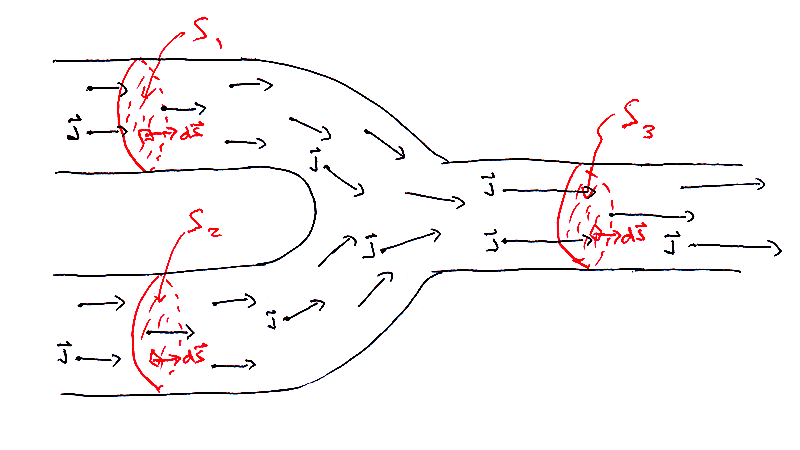# Electric current is not a vector while electric current density is a vector

Delta2
Homework Helper
Gold Member
In order to add them, ##\vec {j_1}## and ##\vec {j_2}## must be evaluated at the same point. How can you do that when they’re in different wires or resistors?
You can add them if you set them to be zero "outside of their domain". But then it is obvious that the equation doesn't hold in all points e.g in a point ##r_2## of the domain of ##J_2## where ##\vec{J_2}(\vec{r_2})\neq\vec{0}## but ##\vec{J}(\vec{r_2})=\vec{J_1}(\vec{r_2})=\vec{0}##

I believe that my example at post #24 shows that even in the junction point that is common to the domain of all three, the equation doesn't necessarily hold.

Dale
Mentor
2020 Award
The reason it doesn't hold necessarily is that the geometry of the circuit around of junction can be anything:
It holds absolutely 100% regardless of the geometry. You are confusing “holds” with “applies”. “Holds” means that the equation is true, and that equation is always true in classical electrodynamics. “Applies” means that it is useful for answering a particular question.

Notice very carefully how the equation we are discussing was originally defined:
if you have two sources of flowing charges at each given point ##\vec{r}## at time ##t## the total current density is ##\vec{j}(t,\vec{r})=\vec{j}_1(t,\vec{r})+\vec{j}_2(t,\vec{r})##.
In contrast your "counter example" is as follows:
Counter example on why it doesn't necessarily hold:

Suppose we have a constant current density ##\vec{J}## with magnitude ##|\vec{J}|=\alpha## that is along the x-axis (it is like a dirac delta function ##\alpha\delta (\sqrt {y^2+z^2})\hat x##) and comes from x<0. At the origin it branches with two branches , one ##\vec{J_1}## along the line y=x (x>0) with magnitude ##\frac{\alpha}{3}## and the other ##\vec{J_2}## along the line y=-x (x>0) with magnitude ##\frac{2\alpha}{3}##. I believe if we do the math at the junction point (the origin) it will be $$\vec{J}(\vec{0})\neq \vec{J_1}(\vec{0})+\vec{J_2}(\vec{0})$$
So in your counter example ##\vec J_1## and ##\vec J_2## are not the current densities due to sources but simply unsourced current densities in the individual wires.

So a proper definition would be ##\vec J_1## is the current density for a source on branch 1, so it includes an amount of current ##\frac{\alpha}{3}## in the x-axis wire, and ##\vec J_2## is the current density for a source on branch 2, so it includes an amount of current ##\frac{2\alpha}{3}## in the x-axis wire. Then indeed $$\vec{J} = \vec{J_1}+\vec{J_2}$$ everywhere including the origin. So it holds but doesn't apply because it already assumes the current bends at the wire which is presumably what you wanted to find out without assuming it.

You cannot change the meaning of the terms and then claim that an equation doesn't hold. If you need to change the meaning of the terms then the equation doesn't apply.

Last edited:
••weirdoguy and Delta2
jtbell
Mentor
The way I picture this is that there is a single ##\vec j## field that is distributed through the interior of the wires and their junction region. We get the different currents in each wire by integrating over different surfaces that span each wire separately.$$\int_{S_1} {\vec j \cdot d \vec S} + \int_{S_2} {\vec j \cdot d \vec S} = \int_{S_3} {\vec j \cdot d \vec S} \\ I_1 + I_2 = I_3$$

•vanhees71, etotheipi and Delta2
Delta2
Homework Helper
Gold Member
It holds absolutely 100% regardless of the geometry. You are confusing “holds” with “applies”. “Holds” means that the equation is true, and that equation is always true in classical electrodynamics. “Applies” means that it is useful for answering a particular question.
Notice very carefully how the equation we are discussing was originally defined:
This is confusing ,in my opinion it doesn't hold neither it applies. However you are right that I had in my mind the equation as it was presented in the context of post #10 and not that of @vanhees71 post.

You cannot change the meaning of the terms and then claim that an equation doesn't hold. If you need to change the meaning of the terms then the equation doesn't apply.
Ok I see now what you meant, we can agree on that.

•Dale
vanhees71
$$\vec{j}(t,\vec{x})=\vec{j}_1(t,\vec{x}) + \vec{j}_2(t,\vec{x}).$$
•